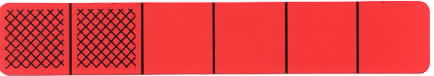Home
 Introducing Fractions Materials: Deck of Fraction Bars and Fraction Playing Cards per group .Ask each group to unbundle a deck of Fraction Playing Cards and make a few observations".Here are a few possibilities: the cards are colored coded to match the bars; and there is a fraction for each bar, including zero bars and whole bars. Point out that the color coding helps to provide a gradual transition to the use of fractions, because the color suggests the number of parts in a bar (denominator) and the student only needs to look at the top number (numerator) of the fraction to determine the number of shaded parts for the bar. Activities for Introducing Fractions "Here are two activities that can be used to introduce fractions to students: I. Bars to Fractions – Selects a bar and then find its card and write its fraction; II. Fractions to Bars – Selects a card and then write a description of its bar. Bars and Cards Bars and Cards is an introductory game to help students make a gradual transition to fraction notation. Layout two bars of each color as shown in the following figure and briefly explain the game of Bars and Cards. The goal is to match a bar to the fraction on the card that has been turned over.Point out that after students have had an extensive period of games and activities with the bars and have described the bars in their own words, they will appreciate using fractions. For example, instead of saying "a red bar with 2 parts shaded" or "a bar with 2 shaded parts out of 6", they can describe the bar as a 2/6 bar and write its fraction.In the following activity the students have placed all the fraction playing cards on a table and are matching each bar with its corresponding fraction.